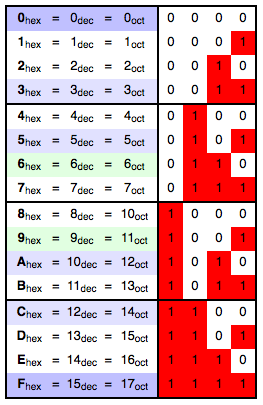As a Decimal to Hex Converter, the Hexadecimal Calculator can convert any decimal figure into a hexadecimal figure and vice versa.

### Convert hexadecimal to decimal number

Put this calculator on your website
• YesNoThe Hexadecimal Calculator converts between Decimals and Hexadecimals. Use the free Hexadecimal Calculator to work out your conversions.

## What is a Hexadecimal Conversion?

The Hexadecimal Calculator converts decimal figures into hexadecimal figures by taking into account the 16 different Hex figures and using them in its programming system. To switch from one system to another,  you can also use the standard conversion table below.## How does the Hexadecimal Calculator work?

The Hexadecimal Calculator offers a simple way of converting between decimal and Hexidecimal figures. Simply fill in the fields of the Hexidecimal Calculator and press the calculate button to recieve the conversion you need.